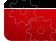首页 | 产品中心 | 联系我们首页 >> 新闻速递

# 纺织常用计算公式

Datetime：2013/9/9 11:21:19   Hits：3727

常州市天祥纺织仪器有限公司

(1)、旦尼尔(D) =g/L*9000 其中g为丝线的重量(克),L为丝线的长度(米)
(2)、特克斯(号数)[tex(H)]: tex=g/L*1000 其中g为纱(或丝)的重量(克),L为纱(或丝)的长度(米)
(3)、分特克斯(dtex): dtex=g/L*9000 其中g为丝线的重量(克),L为丝线的长度(米)

(1)、公制支数(N):N=L/G 其中G为纱(或丝)的重量(克),L为纱(或丝)的长度(米)
(2)、英制支数(S):S=L/(G*840) 其中G为丝线的重量(磅),L为丝线的长度(码)
2、选择换算公式：
(1)、公制支数(N)与旦尼尔(D)的换算公式=9000/N
(2)、英制支数(S)与旦尼尔(D)的换算公式=5315/S
(3)、分特克斯(dtex)与特克斯(tex)的换算公式:1tex=10dtex
(4)、特克斯(tex)与旦尼尔(D)的换算公式:tex=D/9
(5)、特克斯(tex)与英制支数(S)的换算公式:tex=K/S K值:纯棉纱K=583.1 纯化纤K=590.5 涤棉纱K=587.6 棉粘纱(75:25)K=584.8 维棉纱(50:50)K=587.0
(6)、特克斯(tex)与公制数(N)的换算公式:tex=1000/N
(7)、分特克斯(dtex)与旦尼尔(D)的换算公式:dtex=10D/9
(8)、分特克斯(dtex)与英制支数(S)的换算公式: dtex=10K/S K值:纯棉纱K=583.1 纯化纤K=590.5 涤棉纱K=587.6 棉粘纱(75:25)K=584.8 维棉纱(50:50)K=587.0
(9)、分特克斯(dtex)与公制支数(N)的换算公式:dtex=10000/N
(10)、公制厘米(cm)与英制英寸(inch)的换算公式:1inch=2.54cm
(11)、公制米(M)与英制码(yd)的换算公式:1码=0.9144米
(12)、绸缎平方米克重(g/m2)与姆米(m/m)的换算公式:1m/m=4.3056g/m2
(13)、绸缎的实际重量与磅重的换算公式:磅重(lb)=每米绸重(g/m)*0.9144(m/yd)*50(yd)/453.6

 常州市天祥纺织仪器有限公司　版权所有　苏ICP备17045970号 网站技术支持：常州万创网络 Copyright ©　2007-2021　All　Rights　Reserved 在线客户：联系手机：13186660848